# AP Chemistry : Mass Spectroscopy of the Elements

## Example Questions

1 3 Next →

### Example Question #21 : Mass Spectroscopy Of The Elements

What is the correct notation of the isotope of nitrogen with 8 neutrons?

Nitrogen-8

None of these

Nitrogen-15

Nitrogen-7

Nitrogen-14

Nitrogen-15

Explanation:

The denotation is element name-proton+neutron. With 7 protons and 8 neutrons, this necomes Nitrogen-15.

### Example Question #22 : Mass Spectroscopy Of The Elements

A Carbon-12 atom that has gained a neutron and lost an electron can be described as:

a negative ion only

an isotope, and a positive ion.

an isotope only

a positive ion only

an isotope, and a negative ion.

an isotope, and a positive ion.

Explanation:

Isotopes are examples of the same element existing with different amounts of nuetrons.  When an atom gains a neutron, a different isotope of this atom has been formed.  Ions are atoms that have either completely lost or completely gained an electron when compared to their ground state configuration.  Here the atom has lost an electron and is thus a positive ion.

### Example Question #23 : Mass Spectroscopy Of The Elements

Which of the following is a set of isotopes?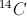and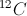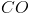and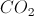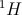and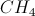and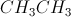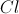and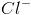andExplanation:

Isotopes are members of the same element that have a different number of neutrons, and therefore a different atomic weight. The number shown in the top left is always the number of protons plus neutrons (atomic weight), so we can show that an element is an isotope by increasing or decreasing that number.

There are three main isotopes of carbon: carbon-12, carbon-13, and carbon-14. The identity of the element must not change, only the atomic weight. The other answer choices are other types of related chemicals; note that ions are not isomers.

### Example Question #51 : Atomic Structure And Properties

An element has two main isotopes. The first isotope has a molar mass of 125 grams, and the second isotope has a molar mass of 128 grams. A naturally occurring sample of the element has a molar mass of 126.8 grams.

Which of the following statements is true concerning the heavier isotope?

It has more protons than the lighter isotope

It has more neutrons than the lighter isotope

It has less neutrons than the lighter isotope

Both isotopes have the same number of nucleons

It has more neutrons than the lighter isotope

Explanation:

When comparing isotopes to one another, remember that they differ by the number of neutrons in the nucleus. A heavier isotope will have more neutrons when compared to a lighter isotope. The number of protons cannot change, as this would change the elemental identity of the compound. The only way to change the atomic mass of an isotope is to change the number of neutrons.

### Example Question #52 : Atomic Structure And Properties

The well-known uranium-235 isotope has how many protons, neutrons, and electrons?

92 protons, 143 neutrons, and 143 electrons

143 protons, 92 neutrons, and 143 electrons

235 protons, 92 neutrons, and 235 electrons

92 protons, 143 neutrons, and 92 electrons

92 protons, 235 neutrons, and 92 electrons

92 protons, 143 neutrons, and 92 electrons

Explanation:

We can start by identifying the atomic number of uranium, which will also be equal to the number of protons. For uranium, the atomic number is 92.

The isotope mass is given in the name of the isotope, in this case uranium-235. The total mass (sum of the protons and neutrons) will be equal to 235.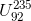To find the number of neutrons, subtract the number of protons from the total mass: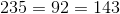.

Finally, for any neutrally charged molecule, the number of electrons will be equal to the number of protons (92).

Our final answer is 92 protons, 143 neutrons, and 92 electrons.

### Example Question #53 : Atomic Structure And Properties

Which isotope of nitrogen has the largest atomic radius?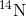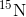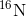The isotopes have the same atomic radius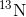The isotopes have the same atomic radius

Explanation:

Atomic radius is generally affected by the interactions of protons and electrons in the atom. Protons attract electrons, condensing the atom, but electrons repel each other, expanding the atom.

Isotopes represent variations in the number of neutrons found in a atom. Neutrons have no charge and neither attract nor repel the electron cloud. As such, increasing the number of neutrons should have no effect on the atomic radius. Each nitrogen isotope has the same atomic radius.

### Example Question #54 : Atomic Structure And Properties

Which of the following describes an isotope to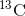?

A carbon with 4 protons, 9 neutrons

A carbon with 8 protons, 5 neutrons

A carbon with 6 protons, 6 neutrons

A carbon atom with 7 protons, 6 neutrons

A carbon with 6 protons, 7 neutrons

A carbon with 6 protons, 6 neutrons

Explanation:

An element is defined by the number of protons it contains. An isotope is the same element with a different number of neutrons; only two choices have six protons, which carbon has. One of them is 13C, which is the same as the question; the other is 12C.

Note that the question asks for an isotope to 13C. By definition, our answer choice cannot be 13C, but must be a variation (an isotope). We are looking for a second answer with 6 protons, in order to maintain the elemental identity of carbon, but a different number of neutrons (not 7). This makes our final answer a carbon atom with 7 protons, 6 neutrons, or 12C, because it is an isotope to 13C.

### Example Question #55 : Atomic Structure And Properties

How many of each subatomic particle exist in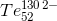?

78 protons, 52 neutrons, 80 electrons

52 protons, 130 neutrons, 54 electrons

52 protons, 78 neutrons, 54 electrons

130 protons, 52 neutrons, 130 electrons

130 protons, 130 neutrons, 132 electrons

52 protons, 78 neutrons, 54 electrons

Explanation:

Using the standard isotope notation of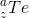, where Z equals the number of protons, and a is the mass number, or the number of protons plus neutrons. Therefore, this isotopic ion has 52 protons and 78 neutrons. Because this anion has a charge of 2-, we know that it contains 54 electrons.

### Example Question #29 : Mass Spectroscopy Of The Elements

Which of the following isotopes of carbon is most abundant on Earth?

Each isotope is equally abundant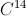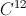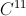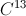We can conclude that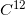is the most abundant carbon isotope.